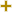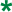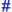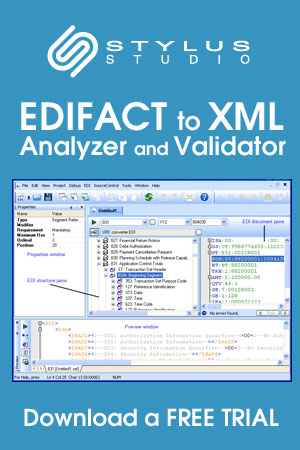# Price multiplier type code qualifierUN/CEFACT Revision 2001A Code ListRepr:an..3
Desc:Code qualifying the type of price multiplier.
This table also occurs in the following versions of this standard:
D00A, D00B, D01A, D01B, D01C, D02A, D02B, D03A, D03B, D04A, D04B, D93A, D94A, D94B, D95A, D95B, D96A, D96B, D97A, D97B, D98A, D98B, D99A, D99B

Coefficient to be used in the price adjustment formula to calculate the revaluated price.
BEscalation coefficient
Coefficient to be used in the escalation formula to calculate the actual price.
CTimesing factor
Factor to be used in calculating the number of times a particular piece of work is repeated.CSD
Cost markup multiplier - original cost
Code specifying the cost mark-up multiplier at original cost.CSR
Cost markup multiplier - retail cost
Code specifying the cost mark-up multiplier at retail cost.DIS
Discount multiplier
Code specifying the discount multiplier.SEL
Selling multiplier
Code specifying the selling multiplier.

Change indicators
plus signAn addition.
asteriskAddition/substraction/change to a code entry for a particular data element.
hash or pound signChanges to names.
vertical barChanges to text for descriptions, notes and functions.
minus signA deletion.
letter XMarked for deletion.

Usage indicatorsUsed in batch messages only.Common usage in both batch and interactive messages.Used in interactive messages only.